Example Questions

← Previous 1 3 4 5 6 7

Example Question #1 : How To Find Synthesis Of Data In Physics

Laura is performing an experiment with a 5kg weight tied to a 3m rope tied to the ceiling as shown: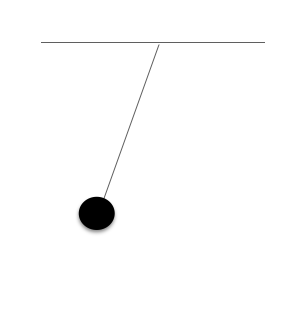Laura drops the weight and allows it to swing freely. She measures how long it takes for the weight to return to it's original position (assume no forces outside of gravity are acting upon the pendulum). This is also called one oscillation.

Experiment 1:

Laura created the following table for her first measurement of the pendulum's oscillations.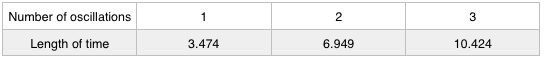Experiment 2:

Laura performed the experiment again, this time using a 6kg weight.Experiment 3:

Laura performed the experiment again, this time using a 3kg weight and a 5m rope.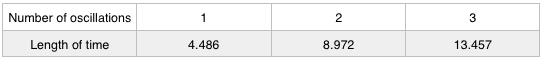How long would 4 oscillations be, using the 3m rope and the 6kg weight?

53.828

13.896

12.566

2.565

13.896

Explanation:

Based on experiment 2, each oscillation is approximately 3.474 seconds long. 4 oscillations would therefore last approximately 13.896 seconds

Example Question #1191 : Act Science

Laura is performing an experiment with a 5kg weight tied to a 3m rope tied to the ceiling as shown:Laura drops the weight and allows it to swing freely. She measures how long it takes for the weight to return to it's original position (assume no forces outside of gravity are acting upon the pendulum). This is also called one oscillation.

Experiment 1:

Laura created the following table for her first measurement of the pendulum's oscillations.Experiment 2:

Laura performed the experiment again, this time using a 6kg weight.Experiment 3:

Laura performed the experiment again, this time using a 3kg weight and a 5m rope.How much longer does each oscillation in experiment 3 take in comparison to experiment 1?

3.14

0.682

1.012

1.111

1.012

Explanation:

To compare how much longer each oscillation in experiment 3 is, simply subtract the two values.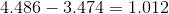Example Question #3 : How To Find Synthesis Of Data In Physics

Laura is performing an experiment with a 5kg weight tied to a 3m rope tied to the ceiling as shown:Laura drops the weight and allows it to swing freely. She measures how long it takes for the weight to return to it's original position (assume no forces outside of gravity are acting upon the pendulum). This is also called one oscillation.

Experiment 1:

Laura created the following table for her first measurement of the pendulum's oscillations.Experiment 2:

Laura performed the experiment again, this time using a 6kg weight.Experiment 3:

Laura performed the experiment again, this time using a 3kg weight and a 5m rope.If Laura recreated experiment 3 using a 5m rope and a 20kg weight, how long would 2 oscillations last?

8.972

6.729

2.243

35.888

8.972

Explanation:

As shown by comparing experiments 1 and 2, the mass of the weight has no effect upon the time of each oscillation.

Example Question #4 : How To Find Synthesis Of Data In Physics

Laura is performing an experiment with a 5kg weight tied to a 3m rope tied to the ceiling as shown:Laura drops the weight and allows it to swing freely. She measures how long it takes for the weight to return to it's original position (assume no forces outside of gravity are acting upon the pendulum). This is also called one oscillation.

Experiment 1:

Laura created the following table for her first measurement of the pendulum's oscillations.Experiment 2:

Laura performed the experiment again, this time using a 6kg weight.Experiment 3:

Laura performed the experiment again, this time using a 3kg weight and a 5m rope.Which of the following could be an equation for the length of time of one oscillation in experiment 1? (L represents the length of the rope)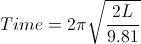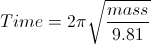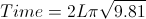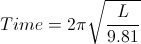Explanation:

By plugging in the values from experiment one, the correct answer can be found.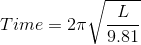Example Question #5 : How To Find Synthesis Of Data In Physics

Laura is performing an experiment with a 5kg weight tied to a 3m rope tied to the ceiling as shown:Laura drops the weight and allows it to swing freely. She measures how long it takes for the weight to return to it's original position (assume no forces outside of gravity are acting upon the pendulum). This is also called one oscillation.

Experiment 1:

Laura created the following table for her first measurement of the pendulum's oscillations.Experiment 2:

Laura performed the experiment again, this time using a 6kg weight.Experiment 3:

Laura performed the experiment again, this time using a 3kg weight and a 5m rope.Laura wants to run a new experiment that has a shorter length of time per oscillation than in experiment 1. Which one of the following would be a good choice for length of rope?

2

3

4

5

2

Explanation:

The shorter the length of rope, the less time each oscillation takes.

Example Question #6 : How To Find Synthesis Of Data In Physics

Laura is performing an experiment with a 5kg weight tied to a 3m rope tied to the ceiling as shown:Laura drops the weight and allows it to swing freely. She measures how long it takes for the weight to return to it's original position (assume no forces outside of gravity are acting upon the pendulum). This is also called one oscillation.

Experiment 1:

Laura created the following table for her first measurement of the pendulum's oscillations.Experiment 2:

Laura performed the experiment again, this time using a 6kg weight.Experiment 3:

Laura performed the experiment again, this time using a 3kg weight and a 5m rope.If Laura recreates experiment 2 using a 300kg weight, how long would each oscillation last?

62.384

3.474

34.74

347.4

3.474

Explanation:

The weight of the object has no effect upon the time of oscillation.

Example Question #7 : How To Find Synthesis Of Data In Physics

Laura is performing an experiment with a 5kg weight tied to a 3m rope tied to the ceiling as shown:Laura drops the weight and allows it to swing freely. She measures how long it takes for the weight to return to it's original position (assume no forces outside of gravity are acting upon the pendulum). This is also called one oscillation.

Experiment 1:

Laura created the following table for her first measurement of the pendulum's oscillations.Experiment 2:

Laura performed the experiment again, this time using a 6kg weight.Experiment 3:

Laura performed the experiment again, this time using a 3kg weight and a 5m rope.If Laura created a new experiment (experiment 4) and used a 3kg mass and a 6m rope, how long would one oscillation likely be?

4.2

4.486

10.123

4.914

4.914

Explanation:

A 6m rope would provide results close to the 5m rope results in experiment 3, but would be slightly bigger. 10 seconds is too long. The other incorrect answers are too small. 4.914 seems most likely.

Example Question #8 : How To Find Synthesis Of Data In Physics

Laura is performing an experiment with a 5kg weight tied to a 3m rope tied to the ceiling as shown:Laura drops the weight and allows it to swing freely. She measures how long it takes for the weight to return to it's original position (assume no forces outside of gravity are acting upon the pendulum). This is also called one oscillation.

Experiment 1:

Laura created the following table for her first measurement of the pendulum's oscillations.Experiment 2:

Laura performed the experiment again, this time using a 6kg weight.Experiment 3:

Laura performed the experiment again, this time using a 3kg weight and a 5m rope.If Laura recreated experiment 1 using a 10kg weight, how long would 2 oscillations last?

10.212

31.416

8.972

6.949

13.898

6.949

Explanation:

As shown by comparing experiment 1 and 2, the actual mass of the weight has no effect upon how long each oscillation is.

Example Question #1192 : Act Science

Laura is performing an experiment with a 5kg weight tied to a 3m rope tied to the ceiling as shown:Laura drops the weight and allows it to swing freely. She measures how long it takes for the weight to return to it's original position (assume no forces outside of gravity are acting upon the pendulum). This is also called one oscillation.

Experiment 1:

Laura created the following table for her first measurement of the pendulum's oscillations.Experiment 2:

Laura performed the experiment again, this time using a 6kg weight.Experiment 3:

Laura performed the experiment again, this time using a 3kg weight and a 5m rope.In experiment 3, how long would 2.5 oscillations last?

16.5

8.5

11.215

13.457

13.5

11.215

Explanation:

Each oscillation lasts 4.486 seconds; therefore, 2.5 oscillations would last 11.215 seconds.

Example Question #10 : How To Find Synthesis Of Data In Physics

Laura is performing an experiment with a 5kg weight tied to a 3m rope tied to the ceiling as shown:Laura drops the weight and allows it to swing freely. She measures how long it takes for the weight to return to it's original position (assume no forces outside of gravity are acting upon the pendulum). This is also called one oscillation.

Experiment 1:

Laura created the following table for her first measurement of the pendulum's oscillations.Experiment 2:

Laura performed the experiment again, this time using a 6kg weight.Experiment 3:

Laura performed the experiment again, this time using a 3kg weight and a 5m rope.Which of the following statements is a valid conclusion?

The length of time of each oscillation is inversely related to the length of the rope

The length of time of each oscillation is inversely related to the mass of the weight

The length of time of each oscillation is directly related to the length of the rope

The length of time of each oscillation is directly related to the mass of the weight